## Sunday, February 23, 2014

### Calculator Art: 2/22/2014

Some calculator art done this week: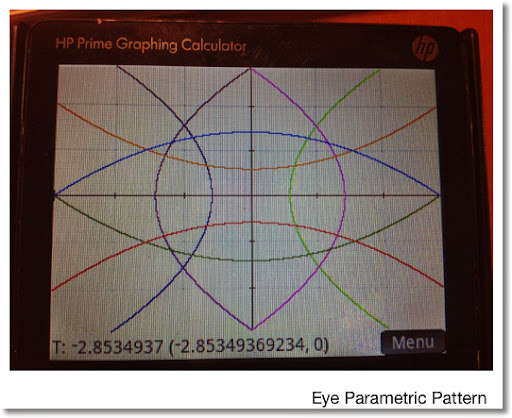Eye Parametric Pattern

HP Prime:

App: Parametric

Range: Autoscale, -2.85349369234 ≤ T ≤ 2.85349369234

Equations:
X1(T) = T
Y1(T) = -0.1736934*T^2 + 1.4142857

X2(T) = T
Y2(T) = -0.1736934*T^2 - 0.587143

X3(T) = T
Y3(T) = -Y1(T)

X4(T) = T
Y4(T) = -Y2(T)

X5(T) = 0.1736934*T^2 - 1.4142857
Y5(T) = T

X6(T) = -X5(T)
Y6(T) = T

X7(T) = 0.17356934*T^2 + 0.5857143
Y7(T) = T

X8(T) = -X7(T)
Y8(T) = T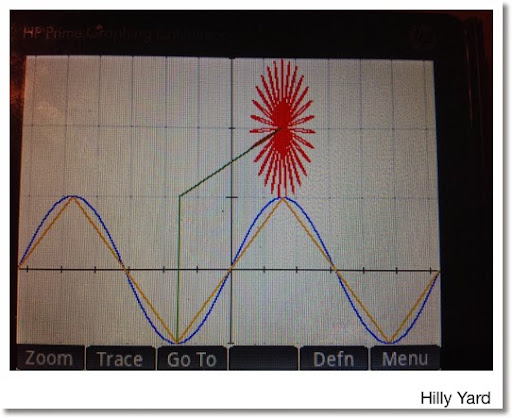Hilly Yard

HP Prime

App: Parametric

Range: -2 π ≤ T ≤ 2 π , Radians Mode

Equations:
X1(T) = T
Y1(T) = PIECEWISE(-2 π ≤ T < -1.5 π, 2T/π + 4,
-1.5 π ≤ T < -0.5 π , -2T/π - 2,
-0.5 π ≤ T < 0.5 π, 2T/π,
0.5 π ≤ T < 1.5 π, -2T/π + 2,
1.5 π ≤ T ≤ 2 π, 2T/π -4)
Color: Orange

X2(T) = T
Y2(T) = sin T
Color: blue

X3(T) = PIECEWISE(-1 ≤ T ≤ 1, -π/2)
Y3(T) = PIECEWISE(-1 ≤ T ≤ 1, T)
Color: Green

X4(T) = PIECEWISE(-π/2 ≤ T ≤ π/2, T)
Y4(T) = PIECEWISE(-π/2 ≤ T ≤ π/2, T/π + 3/2)
Color: Green

X5(T) = 0.15 cos T + π/2
Y5(T) = 0.15 sin T + 2
Color: Red

X6(T) = cos(27*T) sin T + π/2
Y6(T) = cos(27*T) cos T + 2
Color: Red.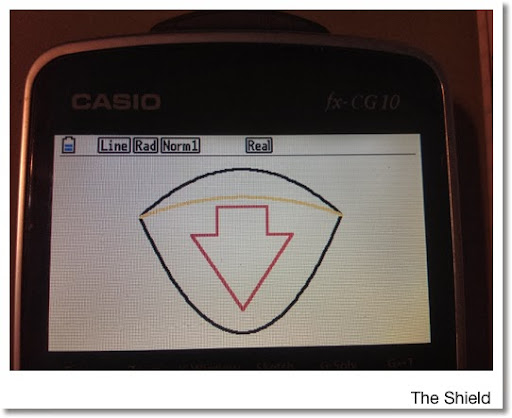The Shield

Casio Prizm

Mode: Graphing

Window:
X = [-3.5, 3.5], Y = [-0.1, 4.5], Range: -2 ≤ T ≤ 2

Equations (Shield):
X1(T) = T
Y1(T) = -0.3125 T^2 + 4.25

X2(T) = T
Y2(T) = 3 T^2 ÷ 4

X3(T) = T
Y3(T) = -0.125 T^2 + 3.5

The arrow was drawn with the FreeLine command.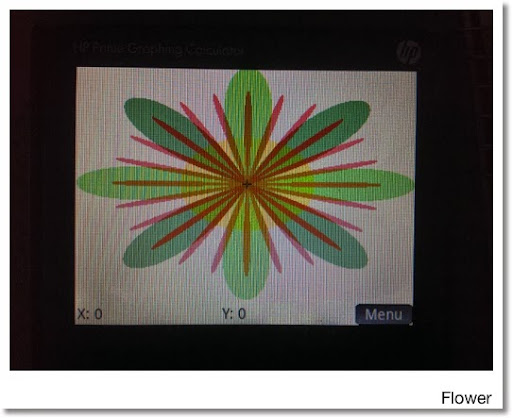Flowers

HP Prime:

Equations:
V1: √(X^2 + Y^2) ≤ 2 cos (24 atan(Y/X))
Color: Red

V2: √(X^2 + Y^2) ≤ 2.5 cos (4 atan(Y/X))
Color: Green

V3: √(X^2 + Y^2) ≤ 2.5 cos (4 (atan(Y/X) - π/4)
Color: Green.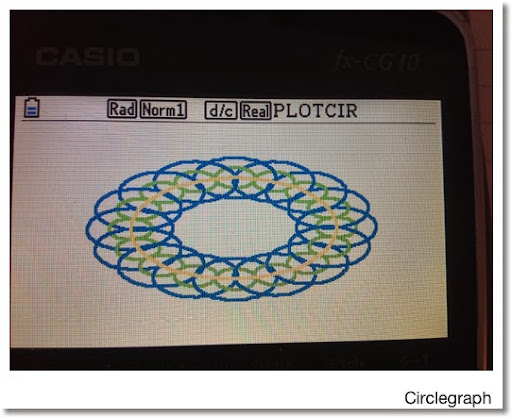Circlegraph

Casio Prizm

Program:
PROGRAM DRAWCIR
Cls
-10 →Xmin
10 → Xmax
-10 → Ymin
10 → Ymax
For 0 → I To 2 π Step π ÷ 8
Plot/Line Color Blue
Circle 5 cos I, 5 sin I, 2
Plot/Line Color Green
Circle 5 cos I, 5 sin I, 1
Next
Plot/Line Color Yellow
Circle 0,0,5

This blog is property of Edward Shore. 2014